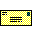# 2016 THEMIS SCIENCE NUGGETS

## On the current density reduction ahead of dipolarization fronts

by San Lu
UCLA EPSS

Introduction

The magnetosphere is drawn out into a long tapered tail due to the interaction between the Earth’s magnetic field and the solar wind. Within this "magnetotail," dipolarization fronts (DFs), transient increases in the northward magnetic field component Bz, have been observed by spacecraft for decades. The interaction of magnetic field fronts and plasma flows with their surrounding current sheets is rather common in geospace, from the solar corona, interplanetary space, to the magnetosphere. DFs can reflect positively charged ions in nearby plasma, creating an intermediate region whose current system is poorly understood. It is important to study this current system because it affects the interaction of the DF with the upstream medium it encounters and the redistribution of the fronts' current system during its propagation. An investigation of the current system interface between DFs and the magnetotail current sheet can improve our understanding of how magnetic energy is converted and electromagnetic waves are generated.

 Figure 1. A long and thin current sheet (white dotted line) is embedded at the equatorial plane of in the magnetotail. A DF (red dashed line) is characterized by a sudden increase of northward magnetic field which propagates towards the Earth (red arrow). The DF is detected by three THEMIS spacecraft whose observations show a current density reduction ahead of the DF. Computer simulations found that the current density reduction is due to the generation of an electrostatic field (shown by the color coded region ahead of the DF, orange means the electric field’s direction is towards north and blue means south) originating from the ion reflection by the DF. Note that the structures’ spatial scale is enlarged for a better illustration. Image Credit: Emmanuel Masongsong, UCLA.

Results

From October to December 2015, three THEMIS spacecraft (TH-A, TH-D, and TH-E) traversed the nightside magnetotail near x = -12RE (GSM coordinates are used throughout this study). The three spacecraft observed a DF with Bz increase from 10 to 20 nT near the equatorial plane (see Figure 2a). Near the beginning of the plotted interval in Fig. 2b, the current density jy in the background current sheet was about 5 nA/m2. Just ahead of the DF, jy dropped to zero; at the DF itself, jy reached 25 nA/m2. The plasma temperature increased and density decreased in the DF region relative to those in the pre-DF region, which is typical in DFs. The plasma velocity Vx shown for TH-E started growing from zero ahead of the DF and reached its maximum value, ~100 km/s, just at the Bz peak. The rise of Vx ahead of the front can be explained by ion reflection from the DF.

 Figure 2. DF observed by three THEMIS spacecraft. (a) Three profiles of the Bz magnetic field (left vertical axis) and ion bulk velocity Vx measured near the neutral plane (right vertical axis). (b) Profiles of current density (black, left vertical axis) and plasma density measured near the neutral plane (red, right vertical axis). (c) Profiles of ion (black, left vertical axis) and electron (green, right vertical axis) temperatures measured near the neutral plane.

A two-dimensional particle-in-cell model was performed to simulate the DF’s interaction with its adjacent plasma and to understand the current density reduction due to the interaction. There is a pair of electric fields Ez ahead of the DF (Figure 3a), which leads to a duskward E × B drift in this region (Figure 3b). The duskward E × B drift, along with a small electron diamagnetic drift, generates a negative electron current, causing the current density reduction ahead of the DF. The simulations further show that the generation of Ez is due to the ion reflection by the DF: ions are reflected and accelerated ahead of the DF, which generates a positive current jx. The ion reflection/acceleration weakens in the region farther away from the DF (Figure 3g), so that a positive charge density is built up by charge conservation (Figure 3f). This charge density generates the Ez.

 Figure 3. The (a) electric field along the z direction, Ez; (b) E × B drift velocity in the y direction; (c) electron diamagnetic drift velocity in the y direction; (d) sum of the electron E × B drift and diamagnetic drift velocities in the y direction; (e) electron bulk velocity in the y direction, Vey; (f) charge density ρ and (g) current density along the x direction, jx. The DF propagates in the direction toward the right. Magnetic field lines (solid lines) are also plotted for reference.

Conclusion

Using THEMIS observations and particle-in-cell simulations, we show that the current density ahead of DFs is significantly reduced relative to the ambient plasma sheet cross-tail current density. The physical mechanism of this reduction is:

1. Ions are reflected and accelerated ahead of DFs, and this reflection causes positive charge density buildup.
2. Positive charge density at the equator generates an electrostatic field Ez directed away from the neutral plane ahead of the DFs.
3. Electron positive (duskward) Ez × Bx drift reduces cross-tail current density. Unlike electrons, ions are demagnetized and do not execute this drift, resulting in a net electron current in the duskward direction that reduces the ambient duskward current.

Reference

Lu, S., A. V. Artemyev, V. Angelopoulos, Q. Lu, and J. Liu (2016), On the current density reduction ahead of dipolarization fronts, J. Geophys. Res. Space Physics, 121, 4269–4278, doi:10.1002/2016JA022754.

Biographical Note

San Lu is an Assistant Researcher in space physics at the University of California, Los Angeles. His primary research interests are computer simulations (particle-in-cell and hybrid) of space and laboratory plasma physics.Please send comments/suggestions to
Emmanuel Masongsong / emasongsong @ igpp.ucla.edu